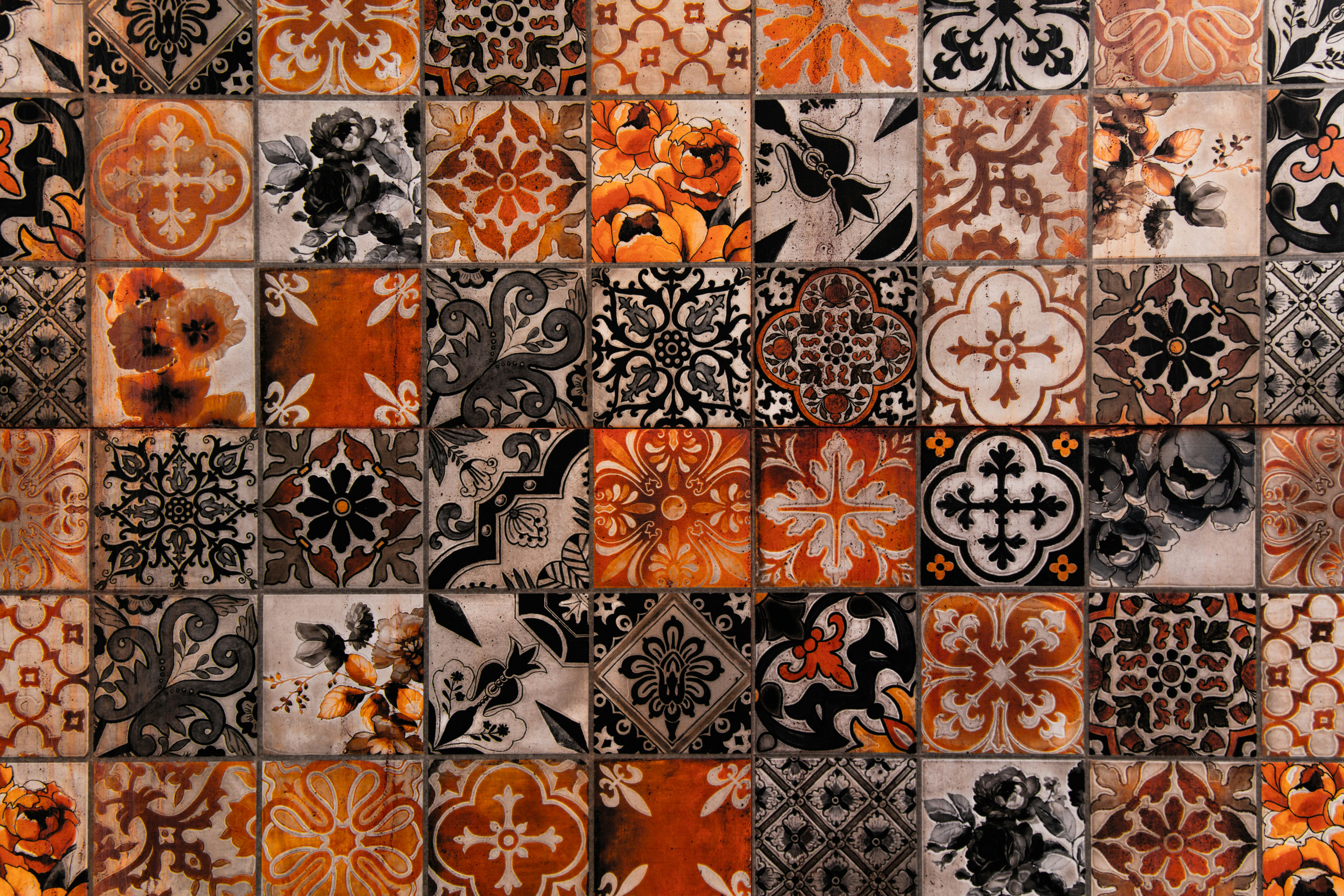Sherlock and Moving Tiles Solution

# Sherlock and Moving Tiles SolutionSherlock is given  square tiles, initially both of whose sides have length  placed in an  plane; so that the bottom left corner of each square coincides with the the origin and their sides are parallel to the axes.

At , both squares start moving along line  (along the positive  and ) with velocities  and .

For each query of form , Sherlock has to report the time at which the overlapping area of tiles is equal to .

Note: Assume all distances in meter, time in seconds and velocities in meter per second unless otherwise specified.

Input Format
First line contains integers . Next line contains , the number of queries. Each of the next  lines consists of one integer  in one line.

Constraints

Output Format
For each query, print the required answer in one line. Your answer will be considered correct if it is at most  away from the true answer. See the explanation for more details.

Sample Input

10 1 2
2
50
100


Sample Output

4.1421
0.0000


Explanation

For the first case, note that the answer is around 4.1421356237..., so any of the following will be accepted:

4.1421356237
4.14214
4.14215000
4.1421
4.1422


### Solution in Python

import math
L,S1,S2 = map(int,input().split())
for i in range(int(input())):
Q = int(input())
H = L-math.sqrt(Q)
R = abs(S1-S2)/math.sqrt(2)
print(H/R)